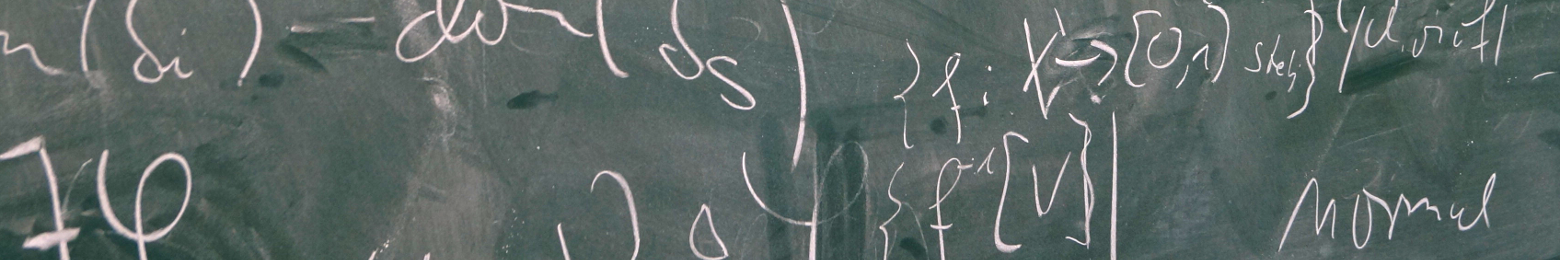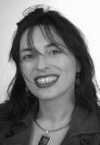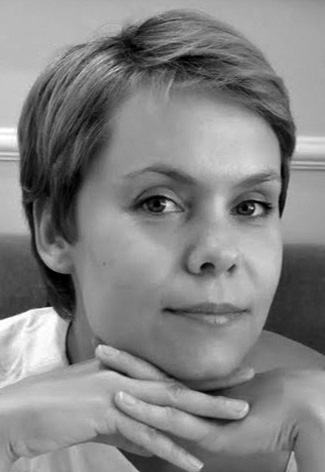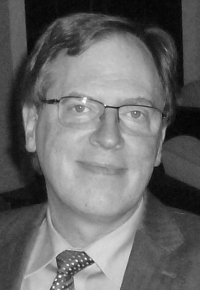## Tutorials

 Mirna Džamonja (University of East Anglia, UK): Independence Results at the Successors of Singular Cardinals The universe of set theory is easy to change by forcing at the successors of regular cardinals, but this is not at all the case at the successors of singulars. This is to do with covering, discovered by Jensen, which says that unless there are large cardinals in the universe, the universe is very close to L and in particular it satisfies the singular cardinal hypothesis. So, even just to have different cardinal arithmetic, we have to assume large cardinals, and if we obtain independence results, the presence of such a large cardinal better be used. In particular, any forcing notion used must necessarily use large cardinals. In the tutorials we shall discuss some known techniques of forcing in these circumstances and will indicate further challenges. SlidesNina Gierasimczuk (DTU Compute, Denmark): Topological Modelling of Knowledge Change Learning can be viewed as a process of adjusting one's knowledge on the basis of observations. So far, combining belief revision procedures with learning-theoretic notions led to many interesting observations about reliability and rationality of mind-change policies within various formal frameworks. In this tutorial we will focus on knowledge change modelled with the use of modal logic (dynamic epistemic logic) and inductive inference (finite and limiting identifiability). General topology allows neat characterisations of many concepts relevant for epistemic logic and inductive inference. In particular, we will see how learnability can be characterised with the use of topological separation properties and we will overview various aspects of employing topological semantics for epistemic logic. Finally, we will browse through open questions concerning the relationships between the two paradigms on topological grounds. Slides (A) Slides (B)Ulrich Kohlenbach (TU Darmstadt, Germany): Proof Mining: Proof Interpretations and Their Use in Mathematics This tutorial gives an introduction to an applied form of proof theory that has its roots in G. Kreisel's pioneering ideas on `unwinding proofs' but has evolved into a systematic activity only during that last 20 years. The general approach is to apply proof theoretic techniques (notably specially designed proof interpretations) to concrete mathematical proofs with the aim of extracting new quantitative results (such as effective bounds) as well as new qualitative uniformity results from (prima facie ineffective) proofs. Logical metatheorems have been developed which guarantee the extractability of highly uniform bounds from large classes of proofs in nonlinear analysis. We will give both (i) an introduction to the underlying proof-theoretic methodology as well as (ii) a survey of some applications to fixed point theory, ergodic theory, optimization and operator theory. Slides Basic Literature: U. Kohlenbach: "Applied Proof Theory: Proof Interpretations and their Use in Mathematics". Springer Monographs in Mathematics, xx+536pp., 2008.Piotr Kowalski (University of Wrocław, Poland): Model Theory of Fields with Operators The aim of my tutorial is to familiarize the audience with the basic notions and ideas of algebraic model theory. I will focus on the model theory of fields with a possible extra structure on a field. In the first hour, I will try to give a friendly introduction to model theory focusing more on concrete examples rather than abstract definitions. I will also show how to prove using model theory some classical results concerning the theory of fields (Ax's theorem about injectivity/surjectivity of polynomials and Hilbert's Nullstellensatz). In the second hour, I will discuss the model theory of fields with operators (derivation/automorphism) and its connections with some problems from diophantine geometry. SlidesMartin Otto (TU Darmstadt, Germany): Bisimulation & Games: Model-Theoretic Aspects Bisimulation is a game-based notion of structural equivalence, which embodies the quintessential format of back & forth equivalences in the model-theoretic tradition of Ehrenfeucht-Fraïssé games. As the name suggests, bi-simulation captures an essentially dynamic, behavioural equivalence between transition systems in terms of a challenge-response probing of possible transitions. In logical terms it captures a natural condition for two states to satisfy exactly the same formulae of (even infinitary) modal logic, whose characteristic quantification pattern also precisely explores the available transitions. In this tutorial I want to give an introduction to bisimulation equivalence, bisimulation games, and their model-theoretic uses in relation to both the classical picture from the perspective of modal logic and to the wider tradition of model-theoretic games starting from Ehrenfeucht-Fraïssé games for classical first-order logic. Among the classical model-theoretic results to be discussed in some detail are van Benthem's characterisation of modal logic as the bisimulation-invariant fragment of first-order logic, as well as some of its extensions and variations that require other than the classical model-theoretic techniques, and put the focus the combinatorics of bisimulation-respecting model constructions. Slides